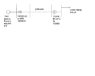# Received voltage, transmission power system

• greg997
In summary, you would choose a voltage level, calculate the current drawn from the load, and then calculate the voltage at the source. You would then compare this voltage to the original source voltage. If deviation is over "acceptable %", you would do another iteration.

#### greg997

Perhaps sb is able to point in the right direction. The task is to calculate the value of the receivig end volatge, for a load of 10 MW at 0.9 pf taken from 66 kV substation.
First I calculated the transmission line impedance
Zlinebase=(Vbase^2)/MVAbase
Zlinebase=132^2/100=174.24Ohm
Zlinepu= 150/174=j0.862
Sbase= 100 MVA
S=10+j4.84
Spu=0.1+j0.048 MVA
T1= j0.1 pu and T2= j0.12 pu
So the total impedace = T1+T2+Line+Load= j0.1+j0.2+j0.862+ 0.1+j0.048= 0.1+j1.21 ohm
Is that correct?
I know that the received volatge is Vr= Vs - Voltage drop. But how can I find the voltage drop? I need current for that, so how to find current?
Any help appreciated.According to MATLAB simulation the received voltage shoud be 57kV Line rms. Is that correct?

#### Attachments

•1.JPG
11.5 KB · Views: 436
Last edited:
Iteration is one simple an accurate way to solve it.

First of all refer all your values to one voltage level.

Assume 66<0 V at the load, calculate backwards to the source, compare your calculated voltage to the original source voltage. If deviation is large, do another iteration with corrected load voltage. Repeat until deviation is acceptable.

Could you please show me how to start with it? If I choose the one voltage level to be 132k then what next? And what do you mean by acceptable deviation? If I got 10900 Vs then is it acceptable?Thank you

There are other methods for solving the problem. If you don't know(or have learned) this method maybe your teacher thinks of another method.

Calculate the current drawn from the load.

Then calculate the voltage at the source; V_calc = V_load + sqrt(3)* Z*I

Compare this voltage to the original source voltage (ref. 66kV) If deviation is over, let's say 5%, do another iteration.